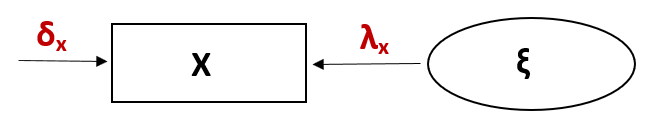# Intoduction to Factor Analysis

In this tutorial, I’ll introduce to you the multivariate statistical method, factor analysis (FA), used for uncovering the underlying relationships between a set of observed variables. It is a widely utilized technique in social and behavioral science for identifying factors such as intelligence, socio-economic status, or personality traits.

Let’s dive into it!

## What is Factor Analysis?

Factor analysis (FA) is a technique used to identify the underlying structure of the data in terms of a smaller set of *unobserved factors (latents). These factors are linear combinations of the *observed variables and help to explain the correlations among them.

This linear relation can be formalized for the observed variable x as x = λx * ξ + δx, where λx refers to factor loadings associated with x, ξ refers to underlying factors, and δx refers to error associated with x. This error is the portion of the variable that can’t be explained by the underlying factors.

The factor analysis model can be visualized in a diagram, where the direction of the causal effect is indicated by arrows. See the figure below.As with all statistical techniques, it relies on some statistical assumptions. Let’s take a look at them!

## Assumptions of FA

It is important that your data meets the following assumptions to obtain reliable results in FA.

• Sufficient Sample Size: Generally, a larger sample size yields more reliable results.
• Linearity: Assumes that the relationship between observed variables and factors is linear.
• Adequacy of Correlations: There should be some correlations between the observed variables, or FA will be ineffective.

Let’s see next what the main FA types and their uses are!

## Main Types of FA

In the realm of FA, two prominent approaches stand out: Exploratory Factor Analysis (EFA) and Confirmatory Factor Analysis (CFA).

• Exploratory Factor Analysis (EFA): EFA is used when the researcher does not have a specific idea of the underlying structure of data. It is employed to explore the possible underlying factor structure without imposing any preconceived structure on the outcome.
• Confirmatory Factor Analysis (CFA): CFA, on the other hand, is used when the researcher has a specific idea of the underlying structure based on theory or previous studies. In CFA, the researcher tests a hypothesized model to see how well it fits the data.

The choice between these two methods should be guided by the specific needs of your study or the research question, allowing for either an exploration of underlying patterns or a focused test of predefined hypotheses.

So far, we have had an overview of the FA concept. Next, we will explore the steps of conducting an FA.

## Steps of FA

• Gather and Prepare Your Data: Collect your dataset and ensure it is cleaned and preprocessed.
• Choose the Number of Factors: Use criteria to decide how many factors to retain, e.g., scree plot.
• Estimate the Factor Model: Use software (like R, SPSS, or Python‘s scikit-learn) to perform FA. The software will estimate the factor loadings (associations between variables and factors), factor variances (variable variance explained by the factors), and unique variances (variable variance not explained by the factors).
• Interpret the Factors: In EFA, examine the factor loadings to interpret what each factor represents; in CFA, check if the variables are associated with the factors as hypothesized. Generally, high loadings (positive or negative) of a variable on a factor indicate strong associations.
• Rotate the Factors (if necessary, in EFA): Rotate results to make the interpretation of factors clearer by simplifying the structure of loadings.
• Confirm the Solution (if necessary, in CFA): Confirm whether the data fits the model well using various fit indices.
• Use the Factors: The factors can be used in further analyses as summary variables or to test hypotheses about the relationships between the factors and other variables.
• Validate Your Model: It’s essential to validate your factor structure on a different sample to ensure that it is generalizable.

## Examples of FA

To learn some more specific information about EFA and CFA, you are welcome to visit our tutorials, Exploratory Factor Analysis and Confirmatory Factor Analysis. However, FA is not limited to these two. There are various specialized or extended types of FA, e.g., Principal Component Analysis, Canonical Factor Analysis.

In this tutorial, we didn’t get into mathematical details, I suggest you check Brown’s book Confirmatory Factor Analysis for Applied Research (2006) for a deeper understanding.

*Observed variables can also be referred to as manifest variables, indicators, and endogenous variables, whereas latent variables can be referred to as factors, constructs, unobserved/underlying variables, and exogenous variables in the context of FA.

## Video, Further Resources & Summary

This tutorial is introductory and simplifies many complex aspects of FA. For deeper study, it is recommended to consult more analysis-specific tutorials.

Do you need more explanations on what the factor analysis is? Then you might check out the following video of the Statistics Globe YouTube channel.

In the video tutorial, we introduce factor analysis as a general concept.

Furthermore, you could have a look at some of the other tutorials on Statistics Globe:

This page was created in collaboration with Cansu Kebabci. You might have a look at Cansu’s author page to get more information about academic background and the other articles she has written for Statistics Globe.

Subscribe to the Statistics Globe Newsletter